# Member CB of the vise shown exerts on block B a force P directed along line CB. Knowing that P must have a 1200-N horizontal component, determine (a) the magnitude of the force P(b) its vertical component.

Question-AnswerCategory: Engineering MechanicsMember CB of the vise shown exerts on block B a force P directed along line CB. Knowing that P must have a 1200-N horizontal component, determine (a) the magnitude of the force P(b) its vertical component.

Member CB of the vise shown exerts on block B a force P directed along line CB. Knowing that P must have a 1200-N horizontal component, determine (a) the magnitude of the force P(b) its vertical component.Step: 1

Draw the free body diagram showing the horizontal and the vertical components of the force P.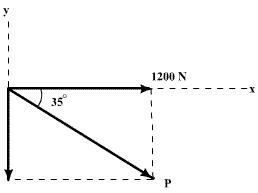Step: 2

(a) The horizontal component of force P is 1200 N. Mathematically, it can be written as follows: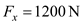Force P makes an angle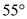with the vertical. Therefore, the angle with respect to the horizontal is obtained as below.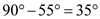From the concept of resolution of forces, the x component of force P is given as follows: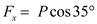Here, the angle is taken as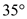with respect to the horizontal.
Substituteforin the equation.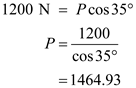Therefore, the magnitude of force P is.
Step: 3

(b) The vertical component of the force P is given as follows:Substitute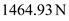for P in the equation.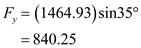Therefore, the magnitude of the vertical component of force P isNow in this question
Pvertical =Psin 35
Phorizontal= 1200N (given)
Pcos35=1200N
P= 1464.9N